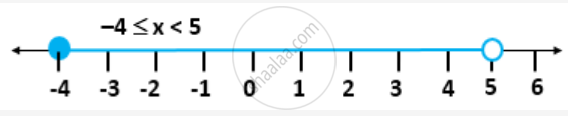Share

# Solve the Following Inequation and Represent the Solution Set on the Number Line 4x - 19 < (3x)/5 - 2 <= (-2)/5 + X, X ∈ R - Mathematics

Course

#### Question

Solve the following inequation and represent the solution set on the number line

4x - 19 < (3x)/5 - 2 <= (-2)/5 + x, x ∈ R

#### Solution

Consider the given inequation

4x - 19 < (3x)/5 - 2 <= (-2)/5 + x, x ∈ R

=> 4x - 19 + 2 < (3x)/5 - 2 + 2 <= (-2)/5 + x + 2, x ∈ R

=> 4x  - 17 < (3x)/5 <= x + 8/5, x ∈ R

=> 4x-(3x)/5<17  "and"  (-8)/5 <=x-(3x)/5,x ∈R

=> (20x  - 3x)/5 < 17 and (-8)/5 <= (5x - 3x)/5, x ∈ R

=> (17x)/5  <  17  and (-8)/5  <= (2x)/5, x ∈ R

=> x/5   < 1 and -4 <= x, x ∈ R

=> x < 5 and -4 <= x, x ∈ R

=> -4 ≤ x < 5; where x ∊ R

The solution set can be represented on a number line as follows:Is there an error in this question or solution?